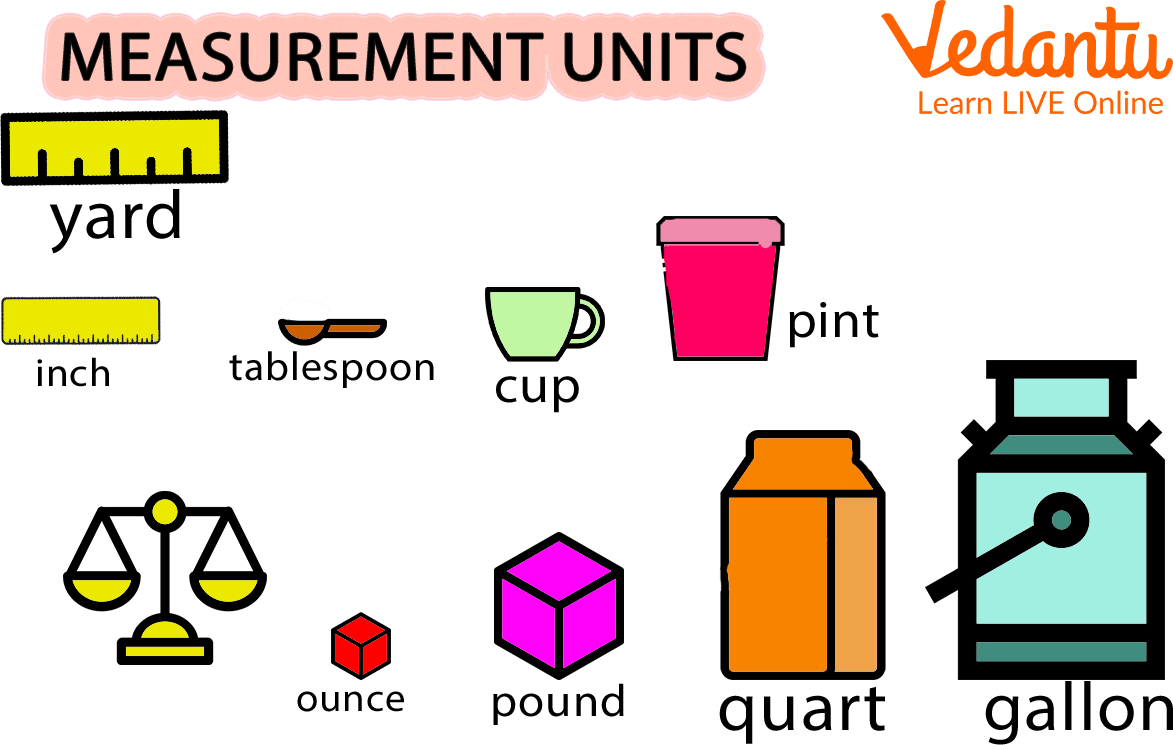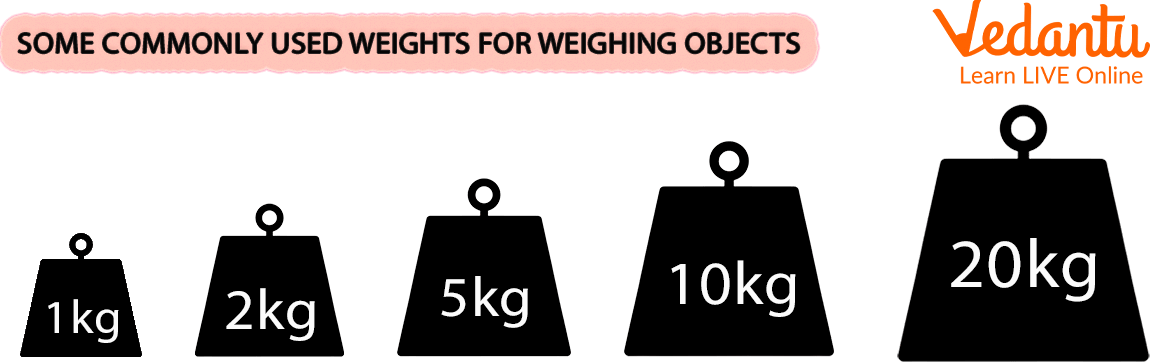Courses
Courses for Kids
Free study material
Free LIVE classes
More

# Weight Measurement UnitsLIVE
Join Vedantu’s FREE Mastercalss

## Introduction to Weight Measurement Units

The Metric System refers to a set of commonly used standard units of measurement.

We are aware that the kilogram, which is abbreviated as "kg," is the primary standard unit of mass or weight. The gram abbreviated "g," is the 1000th part of this kilogram.

So, $1000 \mathrm{~g}=1 \mathrm{~kg}$ and $10000 \mathrm{~g}=10 \mathrm{~kg}$

The gram, which serves as the metric system's unit of mass is very small. We use grams to measure lighter items like popcorn, biscuits, and other snacks. We can use milligrams to quantify objects weighing less than one gram, such as medications $(\mathrm{mg})$. One-thousandth of a gram is equivalent to one milligram.

1000 milligrams $(\mathrm{mg})=1 \operatorname{gram}(\mathrm{g})$

1000 grams $(g)=1$ kilogram $(\mathrm{kg})$

Here, several weight measurements units are explained. Some popular conversions of how to convert 1 quintal in kg and metric ton to kg are also shown.

The weight measurement units are shown below in the picture.Measuring Units

## Weight and Mass

Weight is a measurement of an object's weight. Standard customary units are used to measure weight.

The quantity of material that makes up an object is measured by its mass. Standard metric units are used to measure mass. Kilograms (kg) is the SI unit of mass.

When you're on the earth's surface, the difference is negligible for day-to-day activities. The mass of an object will remain constant when measured on another planet, but its weight will change. (Gravity affects weight, and it varies from world to planet. Because of this, you are weightless when you are in space. However, you still have mass.

## Conversion Units

The International System of Units (SI) prefixes allow weight to be expressed as multiples or fractions of 1 gram:

 1 gigatonne (Gt) $=1000000000000000 \mathrm{~g}$ 1 megatonne (Mt) $=1000000000000 \mathrm{~g}$ 1 tonne (t) $=1000000 \mathrm{~g}$ 1 kilogram (kg) $=1000 \mathrm{~g}$ 1 gram (g) $=1 \mathrm{~g}$ 1 milligram (mg) $=0.001 \mathrm{~g}$ 1 microgram $(\mu g)$ $=0.000001 \mathrm{~g}$ 1nanogram (ng) $=0.000000001 \mathrm{~g}$ 1 picogram (pg) $=0.000000000001 \mathrm{~g}$

Other weights are used to measure the weights of different objects-

Like four $250 \mathrm{gm}$ weights are used which are equal to $1 \mathrm{~kg}$, five $200 \mathrm{gm}$ weights are used which are equal to $1 \mathrm{~kg}$, ten $1000 \mathrm{gm}$ weights are used which are equal to $1 \mathrm{~kg}$.

Therefore, the many units for measuring mass or weight include $1 \mathrm{~kg}, 500 \mathrm{~g}, 250 \mathrm{~g}, 200$ g, $100 \mathrm{~g}, 50 \mathrm{~g}$, etc. The unit weights for measuring $5 \mathrm{~kg}, 10 \mathrm{~kg}, 20 \mathrm{~kg}, 50 \mathrm{~kg}$, and $100 \mathrm{~kg}$ of mass are also available.

## Conversion of Quintal to Kilograms and Tons to Kilograms

In this the conversion of 1 quintal to kg and a metric ton to kg is shown.

One metric tonne is defined as 10 quintal weight.

Consequently, $100 \mathrm{~kg}=1$ quintal and 1 quintal $=100 \mathrm{~kg}$.

10 quintals, or 10 times 100 kilograms, make up one metric tonne.

## Common Balance and Mass Units

With the aid of a balance, the items are weighed. This balance is typically referred to as a common balance. We put the commodities or weight in one pan and the commodities or weight in the other pan.

We can say that there are three primary mass units. We weigh big objects using metric tonnes $(1000 \mathrm{~kg})$ or quintals ( $100 \mathrm{~kg})$, and we use kilograms and grams to measure objects that are used frequently. As a result, extremely heavy objects are measured in quintals and metric tonnes, whereas heavy objects are measured in kilograms, and light ones are measured in grams. Weights of $500 \mathrm{~g}, 250 \mathrm{~g}, 200 \mathrm{~g}, 100 \mathrm{~g}, 50 \mathrm{~g}$, and $25 \mathrm{~g}$ are used.Commonly used Weight

## Solved Examples

Q1. Use specific units: quintal, kg, or g to measure the weights of the following objects:

1. 3 sacks of rice

Ans: Quintal

1. Tin full of vegetable oil

Ans: Kg

1. Spoon

Ans: Grams

Q2. Express 3460 quintal to metric ton.

Ans: One metric tonne is defined as 10 quintal weight.

Therefore, 3460 quintals will be 346 metric tonnes.

Q3. Convert the 4000 grams into kgs.

Ans: 1 kg is 1000 gms

Therefore 4000 gms will be 4 kgs.

## Practice Problems

Q1. Fill ups:

1) 1 quintal = _______ kg

2) 1 metric ton = _______ kg

Ans: 100 kg, 1000 kg

Q2. Convert 5000 quintals to metric tonnes.

Ans: 500 metric tonne

Q3. Convert kgs to grams or vice versa.

1) 6000 gms

2) 3 kgs

3) 4500 gms

Ans: 6 kg, 3000gms, 4.5 kgs

## Summary

Comparing an unknown quantity to a known quantity is referred to as measurement. The measurement yields a numerical value with specific units. Any given object's length, mass, capacity (volume), and temperature can all be measured. A brief knowledge of weight measurement units, conversion units, and common balance and mass units have been discussed here.

Last updated date: 24th Sep 2023
Total views: 124.8k
Views today: 3.24k

## FAQs on Weight Measurement Units

1. Differentiate between mass and weight.

Weight is a measurement of an object's weight. Standard customary units are used to measure weight. SI Unit of weight is Newton.

The quantity of material that makes up an object is measured by its mass. Standard metric units are used to measure mass.  Kilograms (kg) is the SI unit of mass.

Examples: $1 \mathrm{~kg}=1000 \mathrm{gm}, 1 \text { milligram }(\mathrm{mg})=0.001 \mathrm{~g}$

With the aid of a balance, the items are weighed. This balance is typically referred to as a common balance. We put the commodities or weight in one pan and the commodities or weight in the other pan.

2. How do measure weight by common balance?

With the aid of a balance, the items are weighed. This balance is typically referred to as a common balance. We put the commodities or weight in one pan and the commodities or weight in the other pan.

We can say that there are three primary mass units. We weigh big objects using metric tonnes $(1000 \mathrm{~kg})$ or quintals $(100 \mathrm{~kg})$, and we use kilograms and grams to measure objects that are used frequently. As a result, extremely heavy objects are measured in quintals and metric tonnes, whereas heavy objects are measured in kilograms, and light ones are measured in grams. Weights of $500 \mathrm{~g}, 250 \mathrm{~g}, 200 \mathrm{~g}, 100 \mathrm{~g}, 50 \mathrm{~g}$, and $25 \mathrm{~g}$ are used.

3. What are the measurement units used to weigh any object? Change the following $6 \mathrm{~kg}=\ldots \ldots \ldots \ldots . \ldots \mathrm{m} .$

Kilogram, which is abbreviated as "kg," is the primary standard unit of mass or weight. The gram abbreviated "g," is the 1000th part of this kilogram.

So, $1000 \mathrm{~g}=1 \mathrm{~kg}$ and $10000 \mathrm{~g}=10 \mathrm{~kg}$

This unit of mass, the gram $(\mathrm{g})$, is quite tiny. the gram, which serves as the metric system's unit of mass. We use grams to measure lighter items like popcorn, biscuits, and other snacks. We can use milligram to quantify objects weighing less than one gram, such as medications (mg).

$6 \mathrm{~kg}=$ ................... $\text { g }$

Standard unit is $1000 \mathrm{~g}=1 \mathrm{~kg}$

$6 \mathrm{~kg}=6 \times 1000=6000 \mathrm{gm}$

Therefore, $6 \mathrm{~kg}=6000 \mathrm{gms}$